# 1st Order Low-Pass Filter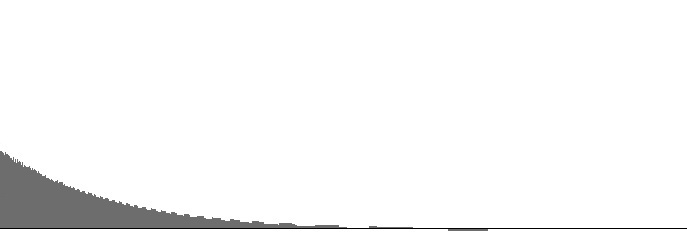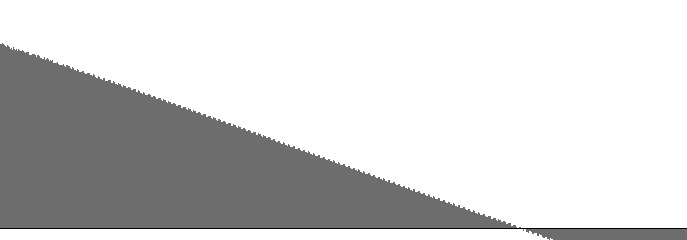Figure 1 (a): FilterLowPass1 amplitude versus log(frequency) with 6 Hz. “cutoff”. Figure 1 (b): FilterLowPass1 log(amplitude) versus log(frequency) with 6 Hz. “cutoff”.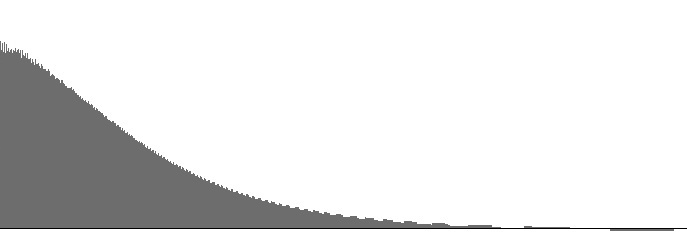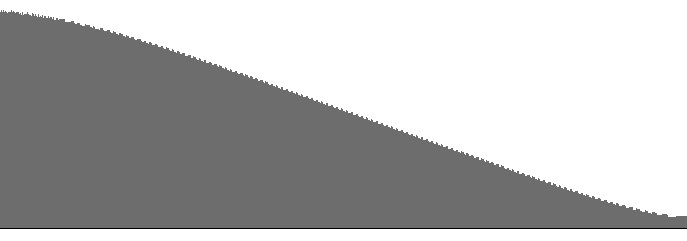Figure 2 (a): FilterLowPass1 amplitude versus log(frequency) with 25 Hz. “cutoff”. Figure 2 (b): FilterLowPass1 log(amplitude) versus log(frequency) with 25 Hz. “cutoff”.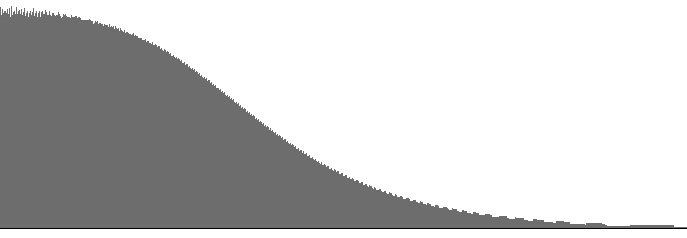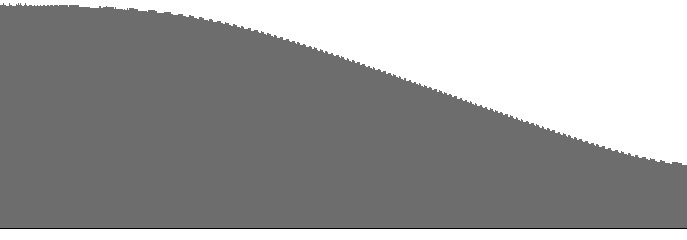Figure 3 (a): FilterLowPass1 amplitude versus log(frequency) with 100 Hz. “cutoff”. Figure 3 (b): FilterLowPass1 log(amplitude) versus log(frequency) with 100 Hz. “cutoff”.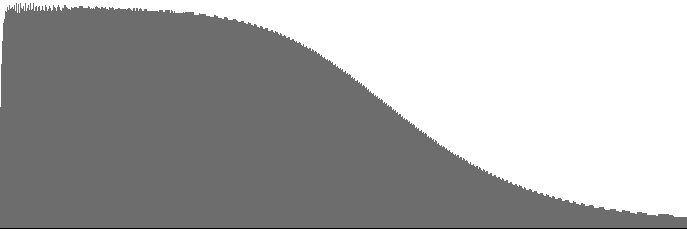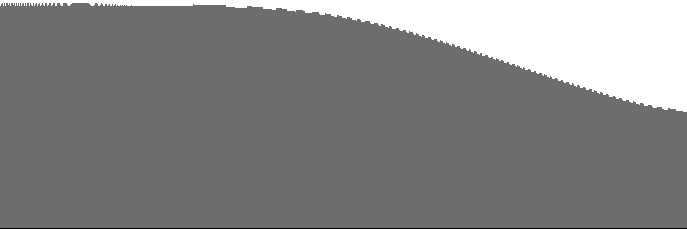Figure 4 (a): FilterLowPass1 amplitude versus log(frequency) with 400 Hz. “cutoff”. Figure 4 (b): FilterLowPass1 log(amplitude) versus log(frequency) with 400 Hz. “cutoff”.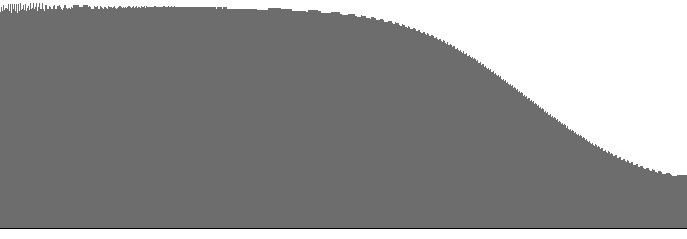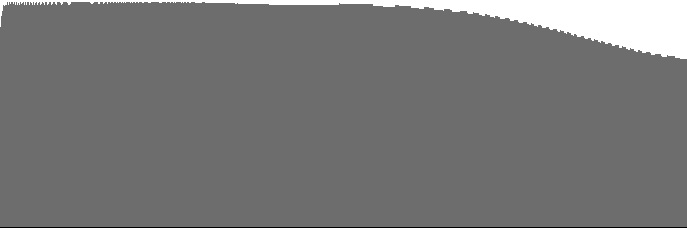Figure 5 (a): FilterLowPass1 amplitude versus log(frequency) with 1600 Hz. “cutoff”. Figure 5 (b): FilterLowPass1 log(amplitude) versus log(frequency) with 1600 Hz. “cutoff”.

The FilterLowPass1 unit implements low-pass filtering with the most gradual dropoff in the suite. Because the dropoff rate is so gradual, the “cutoff” frequency for this filter is much more a guideline than a rule. Figures 1 (a) through 5 (b) plot frequency responses for this unit.

The dropoff rate in decibels per octave is discernable as the slope in the (b) graphs. The steepest dropoff rate is around -2.0 dB per octave; however, notice that this slope appears in all (b) graphs.

One might expect the slope of the (b) graphs to decrease as the cutoff frequency increases, but this is not the case. Rather, there is a region of flat response which first becomes apparent above 25 Hz. and which expands rightward as the cutoff frequency increases. This is in fact the behavior normally expected of a low-pass filter.

Below 25 Hz., differences between frequency-response curves are mostly a matter of gain, so cutoff frequencies below 25 Hz. are not recommended.

 © Charles Ames Page created: 2014-02-20 Last updated: 2015-07-12